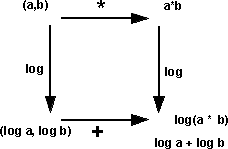# Maths - Lie Algebra

The notation: for Lie multiplication is to put both operands in square brackets:

[x y]

This is known as the lie bracket.

There should only be two terms in the square brackets because the multiplication is not associative.

### Identities

The identities for the lie bracket are:

 [x y] + [y x] = 0 anti-commutative [x [y z]] + [y [z x]] + [z [x y]] = 0 Jacobi identity : anti-associative : anti-symmetry

The first indentity is equivalent to

• [x x] = 0
• and [x y] is bilinear

This equivilance is because:

[x+y x+y] = [x x] + [x y] + [y x] + [y y] = 0

since:

• [x x] = [y y] = 0
• [x y] + [y x] = 0

### Conversion between Lie Algebra and Associative Algebra

We can convert elements of associative algebra to lie algebra by using the bilinear multiplication:

[a,b] = ab - ba

Where:

a,b are elements of an associative algebra

### Geometric Interpretation of Lie Algebra

Tangent spaces of differential manifolds whose points can be multiplied together.

### Example - Vector Cross Product

An example of a Lie algebra is the vector cross product, this applies to vectors in three dimensions.

When we cross multiply two 3D vectors A and B we get:

x = Ay * Bz - By * Az
y = Az * Bx - Bz * Ax
z = Ax * By - Bx * Ay

where:

• Ax,Ay and Az are the components of vector A
• Bx,By and Bz are the components of vector B
• x,y and z are the resulting components

We can summarise the components of this vector cross product in the following multiplication table:

 a×b b.e1 b.e2 b.e3 a.e1 0 e12 -e31 a.e2 -e12 0 e23 a.e3 e31 -e23 0

### The Baker-Campbell-Hausdorff formula

This formula links Lie groups to Lie algebras by expressing the logarithm of the product of two Lie group elements as a Lie algebra.When we are dealing with real numbers we can replace multiplication with addition by taking a logarithm. So can we do this with more complicated quantities shuch as those representing 3 dimensional rotations?

The answer is yes, but its a bit more complicated for this type of quantity. It works well for representing 2D rotations using complex numbers. A rotation of θ can be rpresented by the complex number: cos(θ) + i sin(θ)

To combine two rotations θ1 and θ2 we can multiply the corresponding complex numbers:

(cos(θ1) + i sin(θ1)) * (cos(θ2) + i sin(θ2))

This can be simplified since:

e = cos(θ) + i sin(θ)

so the resulting rotation is:

eiθ1 * eiθ1 = ei(θ1+θ2)

It would be nice if we could do the same for 3D rotations using quaternions, however there is a problem because quaternions and 3D rotations do not commute and so we cannot represent them by addition which is always commutative. We therefore have to use something more complicated than pure addition, we now require an infinite series the first few terms are:

ln(eA eB)

= A + Y + 1/2 [A,B]

+ 1/12 ([A,[A,B]] - [B,[A,B]])

- 1/24 [B,[A,[A,B]]] +...

Where I can, I have put links to Amazon for books that are relevant to the subject, click on the appropriate country flag to get more details of the book or to buy it from them.The Princeton Companion to Mathematics - This is a big book that attempts to give a wide overview of the whole of mathematics, inevitably there are many things missing, but it gives a good insight into the history, concepts, branches, theorems and wider perspective of mathematics. It is well written and, if you are interested in maths, this is the type of book where you can open a page at random and find something interesting to read. To some extent it can be used as a reference book, although it doesn't have tables of formula for trig functions and so on, but where it is most useful is when you want to read about various topics to find out which topics are interesting and relevant to you.